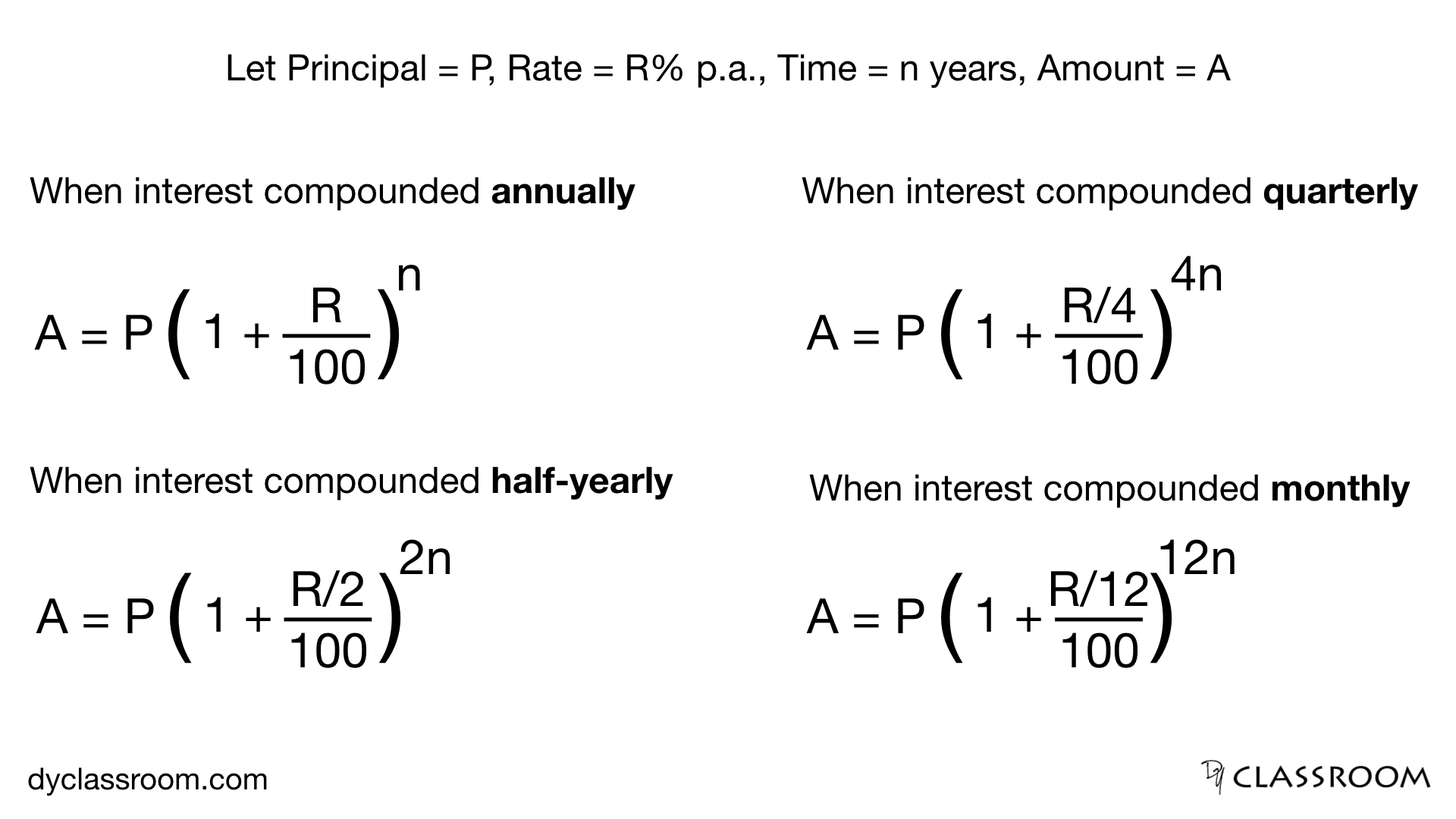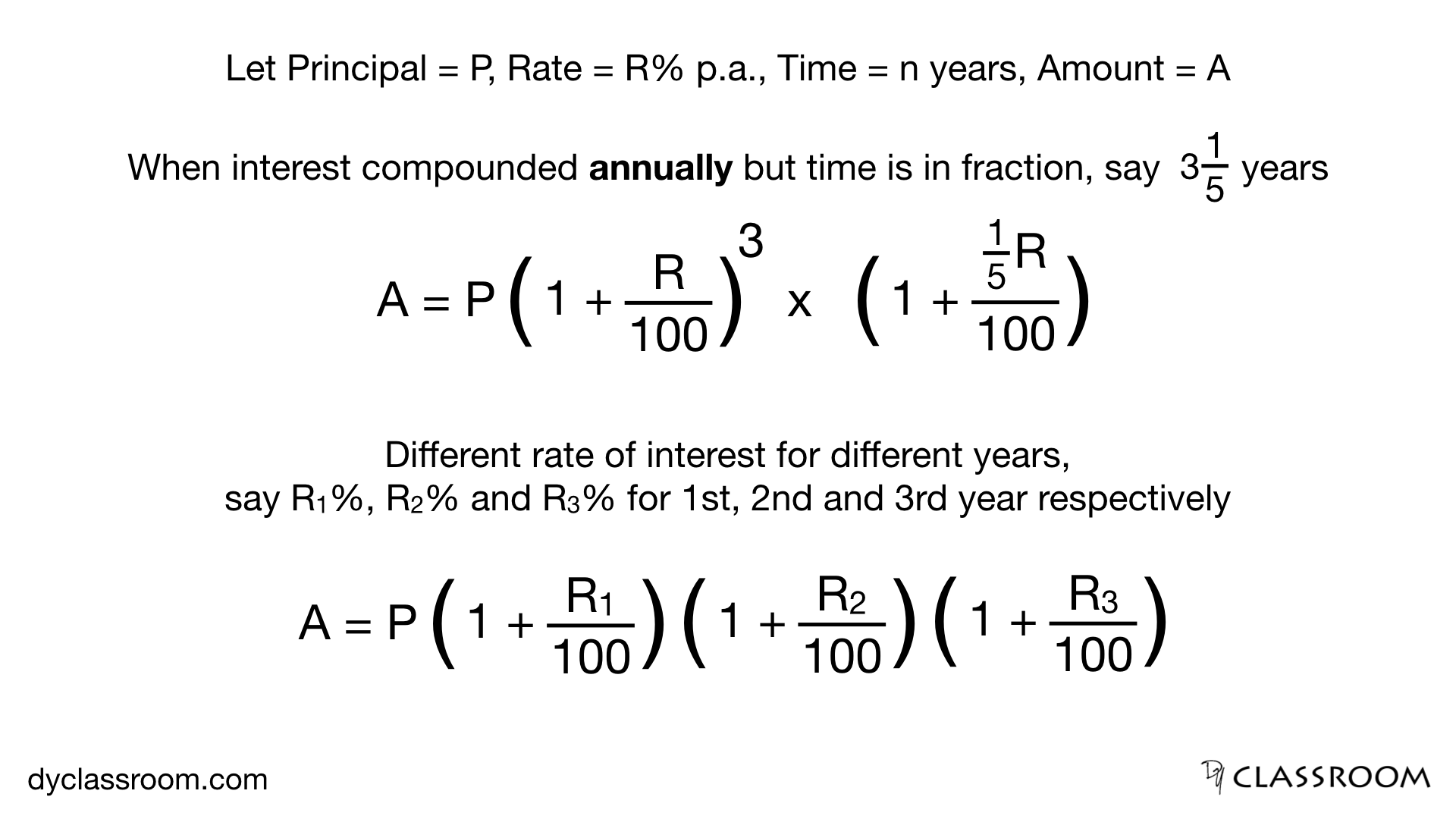# Compound Interest

Aptitude

Compound interest in short is interest on interest.

## Important terms

### Principal

This is the sum of money invested or the amount we borrow from the bank or someone.

### Rate

This represents the rate of interest (generally in per annum). It is used to calculate the interest amount.

### Time

This is the time for which the principal is invested or borrowed.

### Interest

This is the amount that is calculated on the principal using the rate of interest and the time of investment.

Lets take an example to understand compound interest better.

## Example

Lets say we have invested Rs. 1000 at 10% p.a. for 2 years as compound interest.

So, principal P = 1000, rate R = 10 and time n = 2.

Now, lets find the compound interest.

``````1st year
--------------------
Principal for 1st year P = 1000
Rate R = 10

Interest I = PRn/100,    where n = 1 as we are calculating interest for 1 year

or, I = (1000 x 10 x 1) / 100
= 100

Amount at the end of 1st year
A = P + I
= 1000 + 100
= 1100

2nd year
--------------------
Principal for 2nd year = Amount at the end of 1st year
P = 1100

Rate = 10

Interest I = PRn/100,    where n = 1 as we are calculating interest for 1 year

or, I = (1100 x 10 x 1) / 100
= 110

Amount at the end of 2nd year
A = P + I
= 1100 + 110
= 1210
``````

So, after 2 years of investment the principal Rs. 1000 amounts to Rs. 1210.

So, compound interest earned in 2 years on Rs. 1000 at 10% p.a. is (1210 - 1000) i.e. Rs. 210.

Simple Interest for 1 years and Compound interest for 1 year for a given principal and rate of interest are both equal.

## Important formula### Interest compounded annually

This means we are calculating the interest once a year.

### Interest compounded half-yearly

This means we are calculating the interest twice a year.

### Interest compounded quarterly

This means we are calculating the interest 4 times a year.

### Interest compounded monthly

This means we are calculating the interest every month for a year.

## Exercise #1

### Rs. 1000 is invested for 2 years at 10% p.a. compounded annually. Find the compound interest.

``````We have,
Principal P = 1000
Rate R = 10
Time n = 2

Since, the interest is compounded annually
so, we are calculating interest once a year.

Amount A = P ( 1 + R/100 )n
= 1000 ( 1 + 10/100 )2
= 1000 ( 1.1 )2
= 1000 x 1.21
= 1210

So, compound interest CI = A - P
= 1210 - 1000
= 210
``````

So, the compound interest after 2 years of investment is Rs. 210.

## Exercise #2

### Rs. 1000 is invested for 2 years at 10% p.a. compounded half-yearly. Find the compound interest.

``````We have,
Principal P = 1000
Rate R = 10
Time n = 2

Since, the interest is compounded half-yearly
so, we are calculating interest twice a year.

Amount A = P ( 1 + ((R/2) / 100) )2n
= 1000 ( 1 + ((10/2) / 100) )2x2
= 1000 ( 1 + (5/100) )4
= 1000 ( 1.05 )4
= 1000 x 1.21550625
= 1215.51

So, compound interest CI = A - P
= 1215.51 - 1000
= 215.51
``````

So, the compound interest after 2 years of investment is Rs. 215.51.

## Exercise #3

### Rs. 1000 is invested for 2 years at 10% p.a. compounded quarterly. Find the compound interest.

Try this yourself.

## Exercise #4

### Rs. 1000 is invested for 2 years at 10% p.a. compounded monthly. Find the compound interest.

Try this yourself.